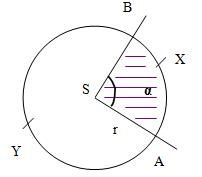# Central angle

What is the length of the arc of a circle with a diameter of 46 cm, which belongs to a central angle of 30°?

Result

x =  24.086 cm

#### Solution:

$D=46 \ \text{cm} \ \\ \ \\ α=30 ^\circ \rightarrow\ \text{rad}=30 ^\circ \cdot \ \dfrac{ \pi }{ 180 } \ =30 ^\circ \cdot \ \dfrac{ 3.1415926 }{ 180 } \ =0.5236=π/6 \ \\ \ \\ x=D \cdot \ α=46 \cdot \ 0.5236 \doteq 24.0855 \doteq 24.086 \ \text{cm}$Our examples were largely sent or created by pupils and students themselves. Therefore, we would be pleased if you could send us any errors you found, spelling mistakes, or rephasing the example. Thank you!

Leave us a comment of this math problem and its solution (i.e. if it is still somewhat unclear...):Be the first to comment!Tips to related online calculators

## Next similar math problems:

1. FlakesA circle was described on the square, and a semicircle above each side of the square was described. This created 4 "flakes". Which is bigger: the content of the central square or the content of four chips?
2. Circular poolThe base of the pool is a circle with a radius r = 10 m, excluding a circular segment that determines the chord length 10 meters. The pool depth is h = 2m. How many hectoliters of water can fit into the pool?
3. Circular segmentCalculate the area S of the circular segment and the length of the circular arc l. The height of the circular segment is 2 cm and the angle α = 60°. Help formula: S = 1/2 r2. (Β-sinβ)
4. DecagonCalculate the area and circumference of the regular decagon when its radius of a circle circumscribing is R = 1mShow that the quadrilateral with vertices P1(0,1), P2(4,2) P3(3,6) P4(-5,4) has two right triangles.
6. NonagonCalculate the area and perimeter of a regular nonagon if its radius of inscribed circle is r = 10cm
7. 30-gonAt a regular 30-gon the radius of the inscribed circle is 15cm. Find the "a" side size, circle radius "R", circumference, and content area.
8. Company logoThe company logo consists of a blue circle with a radius of 4 cm, which is an inscribed white square. What is the area of the blue part of the logo?
9. Quarter circleWhat is the radius of a circle inscribed in the quarter circle with a radius of 100 cm?
10. Circular ringSquare with area 16 centimeters square are inscribed circle k1 and described circle k2. Calculate the area of circular ring, which circles k1, k2 form.
11. SquareCalculate the perimeter and the area of square with a diagonal length 30 cm.
12. The trapeziumThe trapezium is formed by cutting the top of the right-angled isosceles triangle. The base of the trapezium is 10 cm and the top is 5 cm. Find the area of trapezium.
13. WasteHow many percents are waste from a circular plate with a radius of 1 m from which we cut a square with the highest area?
14. Ratio of sidesCalculate the area of a circle that has the same circumference as the circumference of the rectangle inscribed with a circle with a radius of r 9 cm so that its sides are in ratio 2 to 7.
15. Annular areaThe square with side a = 1 is inscribed and circumscribed by circles. Find the annular area.
16. Area of a rectangleCalculate the area of a rectangle with a diagonal of u = 12.5cm and a width of b = 3.5cm. Use the Pythagorean theorem.
17. BillboardRectangular billboard is 2.5 m long with a diagonal 2.8 m long. Calculate the perimeter and the content area of the billboard.